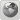Runiter Company
Would you like to react to this message? Create an account in a few clicks or log in to continue.

# Calculator, Memory or Hardware Limitation ? (ln Function)##Calculator, Memory or Hardware Limitation ? (ln Function)

I try to calculate time dilatation via the formula for the example http://de.wikipedia.org/wiki/Zeitdilatation#Reise_zu_entfernten_Sternen

$\tau=\frac{c}{a} \ln \left[\frac{\sqrt{c^2 + [a t + v_0 \gamma_0]^2}+a t + v_0 \gamma_0}{[c + v_0] \gamma_0} \right]$

I brought this to the input form

(2*(c/9.81*ln((√(c^2+(9.81*(1E7)*365*24*3600)^2)+9.81*(1E7)*365*24*3600))/c)+(2*c/(-9.81)*ln((√(c^2+((-9.81)*(1E7)*365*24*3600)^2)+(-9.81)*(1E7)*365*24*3600))/c)+6*30.5*24*3600)/(365*24*3600)

and the calculator is calculationg for hours now, and does not show any result. I expect the output around 56 years in seconds (56*365*24*3600) but I can´t test that because either the hardware is too slow, or the calculator gave up.

I put a screenshot of my hardware here: http://666kb.com/i/byd30f7g5plnnboot.png

I also tried to seperate the calculation but the calculator does not even make
ln((√(c^2+((-9.81)*(1E7)*365*24*3600)^2)+(-9.81)*(1E7)*365*24*3600))/c)
Maybe it is my hardware, if your PC is strong enough please post me the result for the red calculation !

Yukterez

Posts : 22
Join date : 2011-08-03

##Re: Calculator, Memory or Hardware Limitation ? (ln Function)

Sorry I cant modify my posting, I have a mistake (1E7 instead of correctly 1E6 for a million - shame on me)
The correct calculation is therefore:

(2*(c/9.81*ln((√(c^2+(9.81*(1E6)*365*24*3600)^2)+9.81*(1E6)*365*24*3600))/c)+(2*c/(-9.81)*ln((√(c^2+((-9.81)*(1E6)*365*24*3600)^2)+(-9.81)*(1E6)*365*24*3600))/c)+6*30.5*24*3600)/(365*24*3600)

I found a guy who calculated it with an old pocket calculator "SHARP Pocket Computer PC 500S"
http://nunki.de/forum/viewtopic.php?f=6&t=228&p=4237#p4237

So please post me the result for the green calculation instead of the red one if possible, I already put my Percision down to 10 Digits and I still get no result...

Yukterez

Posts : 22
Join date : 2011-08-03

##Re: Calculator, Memory or Hardware Limitation ? (ln Function)

Hi Yukterez

There is indeed a problem with the log and ln functions in Smart Math Calculator that causing it to be ridiculously slow. We tried to make our Logarithm computations super accurate but in the process we made it painfully slow.

This problem will be fixed in the next release 2.3, hopefully within few weeks.

Meanwhile if you reduce the precision to just 2 digits it will give you an answer within 2 minutes. I know 2 digits is extremely inaccurate.
Other than that see if you can avoid using the ln() function.

I will keep you updated about progress in fixing this issue.runiter

Posts : 135
Join date : 2007-12-05
Age : 39
Location : Boston, MA, USAPermissions in this forum:
You cannot reply to topics in this forum